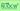# Statistical Analysis Advanced CourseLecturer Takafumi KANAMORI, Associate Professor Graduate School of Information Science, 2016 Spring Department of Computer Science and Mathematical Informatics (2・週1回全15回)

### Course Contents

1. Review of probability theory: probability, conditional probability, expectation value, dispersion and other basics.
2. Linear regression: least-square method, regularization, and cross-validation method.
3. Kernel method: kernel regression analysis, and reproducing kernel Hilbert space.
4. Discriminant analysis: Bayes' rule and bias-variance tradeoff.
5. Support vector machine: algorithm, kernel SVM, and multi-classification.

### Course Features

In order for students to learn smoothly, all materials given in class will be available online. Also, submitted reports will be marked and returned in a week or so to promote self-study. There will be a specific emphasis on mathematical aspects in this class. The aim of this class is to be able to clearly express the train of thought used in information science, which is different from the conventional scientific paradigm.

### Course Aims

To learn theoretical basics of machine learning and mathematical statistics.

### Textbooks

None. Handouts will be provided.

Mohri, et al., Foundations of Machine Learning, The MIT Press, 2012.

### Schedule

Session Contents
1 Introduction, review of probability and statistics
2 Linear regression: least-square method
3 Properties of least-square method and cross-validation method
4 High dimensional model and regularization
5 Kernel regression analysis
6 Positive-definite kernel
7 Reproducing kernel Hilbert space
8 Discriminant analysis
9 Bayes' rule
10 Evaluation of prediction error and bias-variance tradeoff
11 Surrogate loss
12 Support vector machine (SVM): Algorithm
13 Support vector machine (SVM): Statistical property
14 Kernel SVM, multiple variable SVM
15 Multi-class classification based on error correcting output coding (ECOC) method